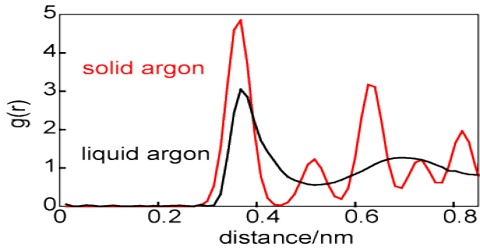Chemistry

# Distribution of Solid between Two Immiscible Liquids: The Distribution LawDistribution of a Solid between Two Immiscible Liquids: The Distribution Law

Iodine dissolves in water only slightly to form a pale yellow solution. If some solid potassium iodide, KI, is added to the solution it turns deep brown and the iodine dissolves. The color is due to the formation of I3 ion which is deep brown in solution. Now some carbon tetrachloride is added to the solution. Water and carbon tetrachloride being immiscible with each other the two liquids form separate layers. If the mixture of the two liquids is now shaken and then allowed to settle down it will be found that the carbon tetrachloride layer has become violet. This is because iodine dissolves in carbon tetrachloride. Thus iodine has been extracted by the organic layer from the aqueous layer.

In general it has been found that when a solute is shaken up with two liquids which are immiscible with each other, but in both of which the solute is soluble, then the solute distributes itself between the two liquids in such a way that the ratio of the concentrations of the solute in the two liquids is a constant at a constant temperature, provided the solute is in the same molecular condition in the two liquids.

This is the statement of what is known as Nernst Distribution Law. If C1 and C2 are the concentrations of this solute in solvent ‘1’ and solvent ‘2’ respectively, then according to this law;

C1/C2 = KD = Constant

The ratio KD is known as the Distribution Coefficient or Partition Co-efficient. As we know in the third form of Henry’s law is another way of stating the distribution law. The statement of Nernst distribution law came long after Henry’s law was stated, indicating that the full significance of Henry’s law was not understood earlier.